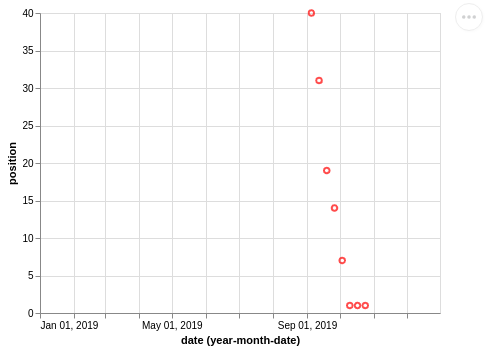# Python: Altair - Setting the range of Date values for an axis

In my continued experiments with the Altair visualisation library, I wanted to set a custom range of data values on the x axis of a chart. In this blog post we’ll learn how to do that.

We’ll start where we left off in the last blog post, with the following code that renders a scatterplot containing the chart position of a song on a certain date:

``````import altair as alt
import pandas as pd
import datetime

df = pd.DataFrame( [
{"position": 40, "date": datetime.date(2019,9,5)},
{"position": 31, "date": datetime.date(2019,9,12)},
{"position": 19, "date": datetime.date(2019,9,19)},
{"position": 14, "date": datetime.date(2019,9,26)},
{"position": 7, "date": datetime.date(2019,10,3)},
{"position": 1, "date": datetime.date(2019,10,10)},
{"position": 1, "date": datetime.date(2019,10,17)},
{"position": 1, "date": datetime.date(2019,10,24)},
])
df["date"] = pd.to_datetime(df["date"])

chart = alt.Chart(df).mark_point(color="red").encode(
x='date',
y='position')
chart.save("chart.html")``````

If we run this script, we’ll have the following chart in `chart.html`:Figure 1. Chart showing chart positions

At the moment the x axis of our chart covers the range of dates included in our DataFrame. I wanted to have that axis start from 1st January 2019 and end on 31st December 2019, and came across a GitHub issue describing how to do this.

One way to do this is to create a Pandas index and then define a custom domain for the `scale` of the x axis. The code below does this:

``````domain_pd = pd.to_datetime(['2019-01-01', '2019-12-31']).astype(int) / 10 ** 6
chart = alt.Chart(df).mark_point(color="red").encode(
x=alt.X('date:T', timeUnit='yearmonthdate', scale=alt.Scale(domain=list(domain_pd))),
y='position')
chart.save("chart.html")``````

If we run this script, we’ll have the following chart in `chart.html`:Figure 2. Chart showing chart positions

Another, simpler way, is to pass in a list containing the start and end dates, as shown below:

``````domain = ["2019-01-01", "2019-12-31"]
chart = alt.Chart(df).mark_point(color="red").encode(
x=alt.X('date:T', timeUnit='yearmonthdate', scale=alt.Scale(domain=domain)),
y='position')
chart.save("chart.html")``````

All the code from this blog post is available in a GitHub Gist.